## Estimator

An estimator is a rule that tells how to calculate an Estimate based on the measurements contained in a sample. For example, the sample Mean'' Average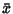is an estimator for the population Mean.

The mean square error of an estimator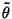is defined by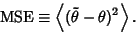Letbe the Bias, then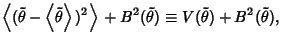where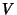is the estimator Variance.